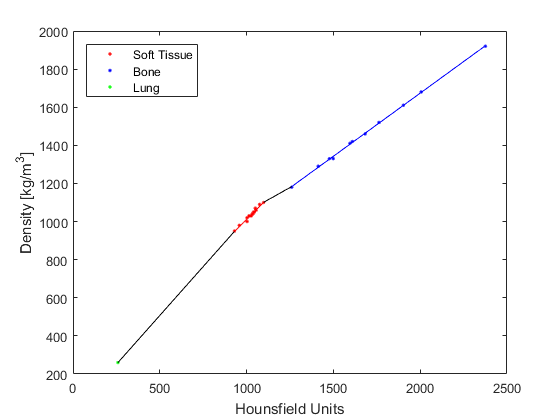hounsfield2density

Convert Hounsfield units to density.

Syntax

density = hounsfield2density(ct_data)
density = hounsfield2density(ct_data, plot_fitting)
[density, sound_speed] = hounsfield2density(ct_data)
[density, sound_speed] = hounsfield2density(ct_data, plot_fitting)

Description

hounsfield2density converts Hounsfield units to units of density [kg/m^3] based on the experimental data given by Schneider, U., Pedroni, E., and Lomax A., "The calibration of CT Hounsfield units for radiotherapy treatment planning," Phys. Med. Biol., 41, pp. 111-124 (1996). The conversion is made using a four-part piece-wise linear fit to the data (see figure below). For soft-tissue, the approximate sound speed can also be returned using the empirical relationship given by Mast, T. D., "Empirical relationships between acoustic parameters in human soft tissues," Acoust. Res. Lett. Online, 1(2), pp. 37-42 (2000).Inputs

 ct_data CT data in Hounsfield units to convert to density

Optional Inputs

 plot_fitting Boolean controlling whether the original data points and fitting is plotted (default = false)

Outputs

 density density in kg/m^3 sound_speed sound speed in m/s